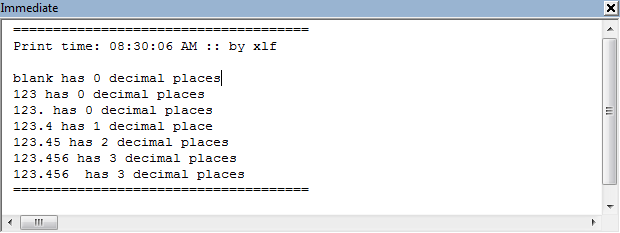xlf QandA series

How to count the number of decimal places in a text string

QUESTION

I WANT TO FORMAT A USERFORM TEXTBOX CONTROL. HOW DO I COUNT THE NUMBER OF NON BLANK CHARACTERS AFTER THE DECIMAL POINT?Answer

Let the decimal separator be the decimal point ".". Code 1 contains a user defined named GetDPstr that returns the number of decimal places in a text string. This is achieved by counting the number of non blank characters after the decimal separator.

Selected VBA functions used in this module.

VBA functions Description
InStr([start], string, substring, [compare])Returns an integer specifying the start position of the first occurrence of a substring within a string
Len(text)Returns an integer value representing the number of characters in a string.
Replace(string, find, replacement, [start, [count, [compare]]])Replaces a sequence of characters in a string with another set of characters
Rtrim(text)Removes trailing spaces from the end (right) of a string

The GetDPstr function

Here is the code logic for the GetDPStr function.

In code 1, line 3 - determine if the string strIn contains a decimal place - by comparing its original length, using the Len function, to its length after "." is replaced by "", using the Replace function. A difference of 0 indicates that strIn does not have a decimal place "." character, thus GetDPstr = 0 in line 4.

Else, line 6 - remove any trailing space characters using the RTrim function, then find the position of the "." character with the InStr function. For example $123.456$ has 7 characters, Len returns 7, and InStr("123.456",".") returns 4. Thus, the number of decimal places is 7 - 4 = 3, and GetDPstr = 3

Code 1: The GetDPstr function: get the number of decimal places in a text string
Function GetDPstr(strIn As String) As Integer
' Returns for the number of decimal places in a string
If Len(strIn) - Len(Replace(strIn, ".", "")) = 0 Then ' test for decimal point separator
GetDPstr = 0
Else
GetDPstr = Len(RTrim(strIn)) - InStr(RTrim(strIn), ".")
End If
End Function


Testing

Test data shown in code 2 includes a space "" at line 4; integer, line 5; and a trailing space, line 10. The Debug.Print statement sends the output to the immediate window.

Code

Code 2: A macro for testing the GetDPstr function. Output is sent to the VBE immediate window.
Sub TestGetDPstr()
Debug.Print "====================================="
Debug.Print "Print time: " & Format(Time, "HH:MM:SS AM/PM") & " :: by xlf" & vbNewLine
Debug.Print "blank" & " has " & GetDPstr("") & " decimal places"            ' returns 0
Debug.Print "123" & " has " & GetDPstr("123") & " decimal places"           ' returns 0
Debug.Print "123." & " has " & GetDPstr("; 123#; ") & " decimal places"     ' returns 0
Debug.Print "123.4" & " has " & GetDPstr("123.4") & " decimal place"        ' returns 1
Debug.Print "123.45" & " has " & GetDPstr("123.45") & " decimal places"     ' returns 2
Debug.Print "123.456" & " has " & GetDPstr("123.456") & " decimal places"   ' returns 3
Debug.Print "123.456 " & " has " & GetDPstr("123.456 ") & " decimal places" ' returns 3
Debug.Print "====================================="
End Sub


Immediate windowFig 1: The immediate window - with Debug.Print values from code 2
• This example was developed in Excel 2013 Pro 64 bit.